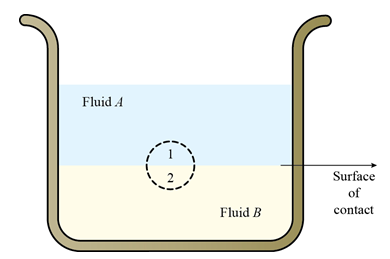×#### Thank you for registering.

One of our academic counsellors will contact you within 1 working day.

Click to Chat

1800-1023-196

+91-120-4616500

CART 0

• 0

MY CART (5)

Use Coupon: CART20 and get 20% off on all online Study Material

ITEM
DETAILS
MRP
DISCOUNT
FINAL PRICE
Total Price: Rs.

There are no items in this cart.
Continue Shopping
```
The surface of contact of two fluids of different densities that are at rest and do not mix is horizontal. Prove this general result (a) from the fact that the potential energy of a system must be a minimum in stable equilibrium, (b) from the fact that at any two points in a horizontal plane in either fluid the pressures are equal.
The surface of contact of two fluids of different densities that are at rest and do not mix is horizontal. Prove this general result (a) from the fact that the potential energy of a system must be a minimum in stable equilibrium, (b) from the fact that at any two points in a horizontal plane in either fluid the pressures are equal.

```
5 years ago396 Points
```							(a)Let us consider two fluids A and B having different densities in a container.It is shown below:Here, fluid A is denser than fluid B .As density is directly related to mass, fluid A will have larger mass than fluid B .Imagine an infinitesimally small sphere region where equal volumes of the two fluids are in contact. It is assumed to be formed by the hemispheres 1 and 2. But the hemisphere 1 has larger mass than 2. Thus, hemisphere 1 can be considered to superimpose with additional mass. The orientation of the hemisphere 1 is the only variable when calculating the potential energy. When the surface of contact is horizontal between the fluids, the center of mass of the hemisphere 1 will be a low as possible. This makes the potential energy at the contact surface to be equal for both the fluids.Therefore, the fluid interfaces will be horizontal.(b)Consider the surface of contact between the two fluids is not horizontal. As the pressure depends on the depth of the fluid, there will be two different values for the pressure difference on the corresponding two points on the surface of contact. This makes both fluids to flow along the surface of contact. Such flow is equalized when the pressure between the points on the surface of contact are equal. This is possible only when the contact surface is horizontal.Therefore, the fluid interfaces will be horizontal to maintain pressure difference at all point on the surface of contact.
```
5 years ago
Think You Can Provide A Better Answer ?

## Other Related Questions on Mechanics

View all Questions »### Course Features

• 101 Video Lectures
• Revision Notes
• Previous Year Papers
• Mind Map
• Study Planner
• NCERT Solutions
• Discussion Forum
• Test paper with Video Solution### Course Features

• 110 Video Lectures
• Revision Notes
• Test paper with Video Solution
• Mind Map
• Study Planner
• NCERT Solutions
• Discussion Forum
• Previous Year Exam Questions